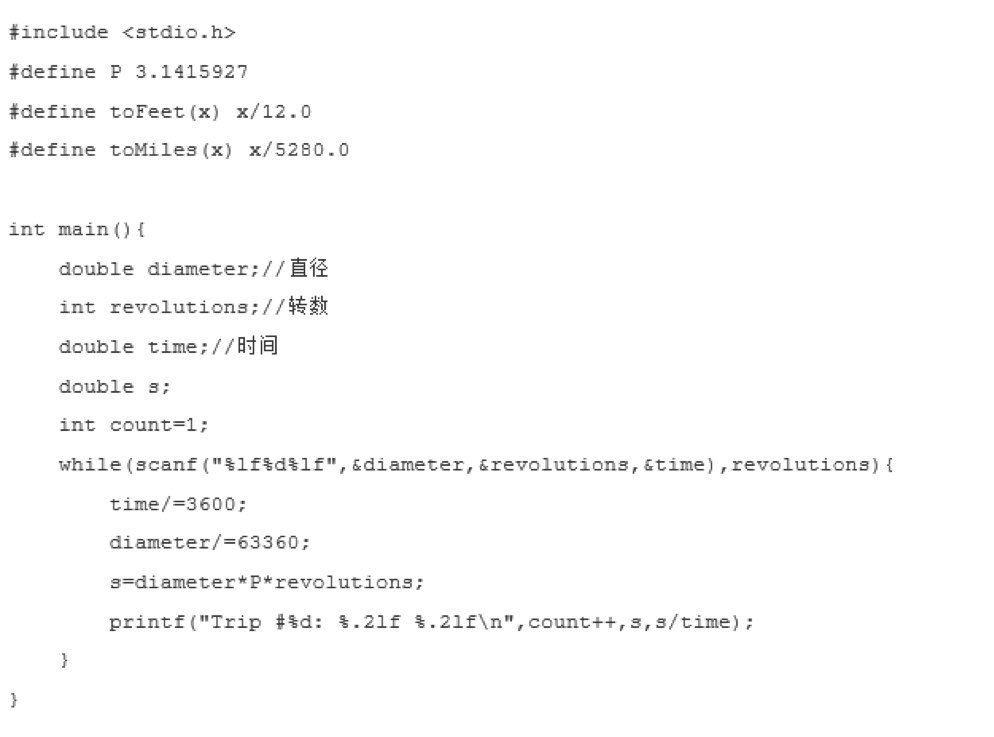2016-05-19 08:57

# 求这段c语言的代码换成java的代码

#include #define P 3.1415927#define toFeet(x) x/12.0#define toMiles(x) x/5280.0int main(){ double diameter;//直径 int revolutions;//转数 double time;//香蕉 double s; int count=1; while(scanf("%lf%d%lf",&diameter,&revolutions,&time),revolutions){ time/=3600; diameter/=63360; s=diameter*P*revolutions; printf("Trip #%d: %.2lf %.2lf\n",count++,s,s/time); }}• 写回答
• 好问题 提建议
• 关注问题
• 收藏
• 邀请回答

#### 2条回答默认 最新

•Monsieur_Zhu 2016-05-19 09:49
已采纳

稍有改动

 import java.util.Scanner;

public class Main {
public static void main(String[] args) {
final double PI = 3.1415927;
Scanner scan = new Scanner(System.in);//标准输入流
int a = scan.nextInt();//首先输入有多少组数据
double x[][] = new double[a];//用二维数组存放数据 直径 转数 时间 依次输入就可以 空格 回车都可以分隔
for (int i = 0; i < x.length; i++) {
for (int j = 0; j < x[i].length; j++) {
x[i][j]=scan.nextLong();
}
}
scan.close();//关闭标准输入流
int count = 1;
for (int i = 0; i < x.length; i++) {
x[i] /= 3600;
x[i] /= 63360;
double s = x[i]*PI*x[i];
System.out.println(String.format("Trip #%d: %.21f %.21f", count++,s,s/x[i]));
}
}
public static double toFeet(double x){//进行换算的方法 传入x返回换算的值 调用方法toFeet(x);
return x/12.0;
}
public static double toMiles(double x){
return x/5280.0;
}
}

已采纳该答案
评论
解决 无用
打赏 举报
•Royal_lr 2016-05-19 09:17

Scanner scan=new Scanner(System.in);
String str=scan.next();
直接接收控制台传过来的数据就行了

评论
解决 无用
打赏 举报